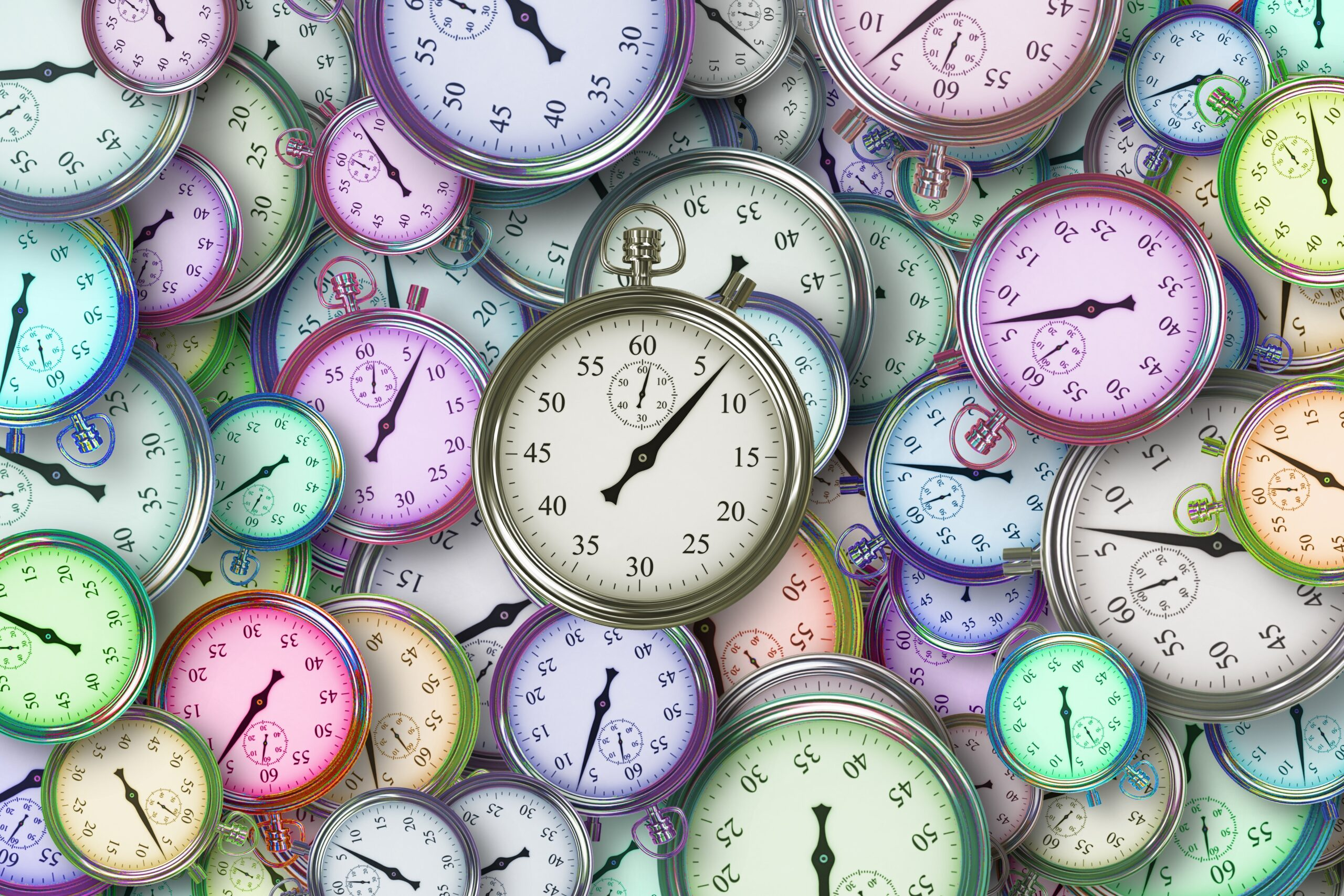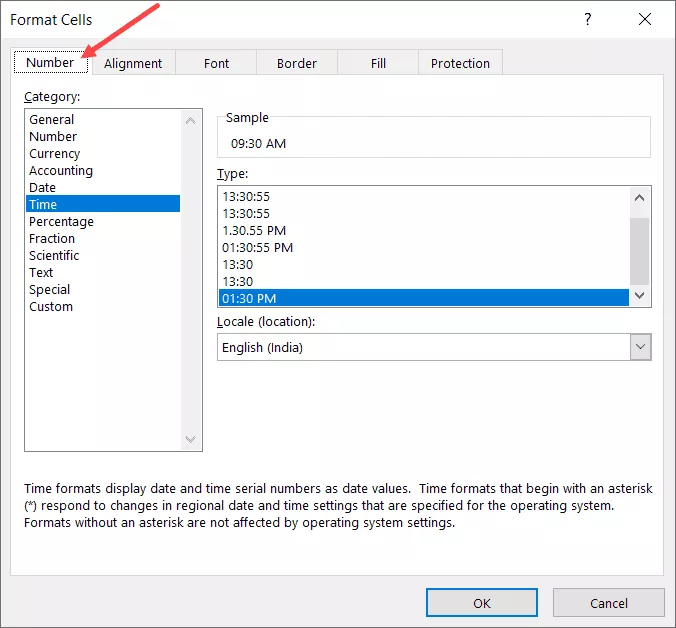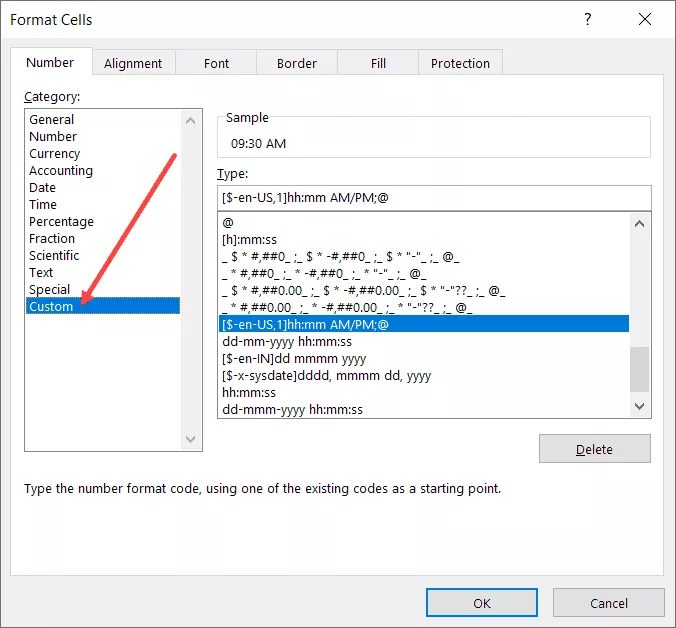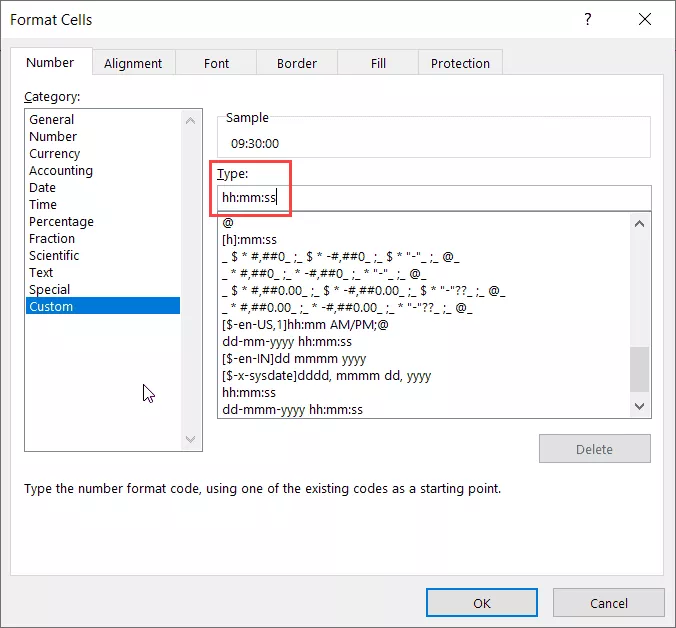# 在 Excel 中計算時間（時差、工作時間、加/減）

## Excel 如何處理日期和時間？

Excel 讓您可以靈活地將小數部分轉換為不同的格式，例如僅小時、僅分鐘、僅秒或小時、分鐘和秒的組合

## 計算兩次時間差的公式

### Excel中計算時差的簡單減法

`結束時間 - 開始時間`

1. 選擇要應用日期格式的單元格
2. 按住 Control 鍵並按 1 鍵（如果使用 Mac，則按 Command + 1）
3. 在打開的“單元格格式”對話框中，單擊“數字”選項卡（如果尚未選擇）4. 在左側窗格中，單擊自定義5. 在類型字段中輸入任何所需的格式代碼（在此示例中，我使用的是 hh:mm:ss）6. 點擊確定

### 以小時、分鐘或秒為單位計算時差

#### 以小時為單位計算時差

`=(B2-A2)*24`

`=INT((B2-A2)*24)`

#### 以分鐘為單位計算時差

`=(B2-A2)*24*60`

#### 以秒為單位計算時差

`=(B2-A2)*24*60*60`

### 使用 TEXT 函數計算時間差

TEXT 函數允許您在公式中指定格式。

`=TEXT（結束日期 - 開始日期，格式）`

`=TEXT(B2-A2,"hh")`

`=TEXT(B2-A2,"[mm]")`

`=TEXT(B2-A2,"[ss]")`

`=TEXT(B2-A2,"[hh]:mm")`

`=TEXT(B2-A2,"hh:mm:ss")`

### 獲取一個單位（小時/分鐘）的時差，忽略其他

`=HOUR(B2-A2)`

`=MINUTE(B2-A2)`

`=SECOND(B2-A2)`

• 結束時間和開始時間之間的差異不能為負數（日期更改時通常會出現這種情況）。在這種情況下，這些公式將返回 #NUM！錯誤
• 這些公式僅使用結果時間值的時間部分（並忽略天部分）。因此，如果結束時間和開始時間的差是 2 天、10 小時、32 分鐘和 44 秒，則 HOUR 公式將給出 10，MINUTE 公式將給出 32，SECOND 公式將給出 44

### 計算到現在經過的時間（從開始時間開始）

NOW 函數返回使用它的單元格中的當前日期和時間。它是不接受任何輸入參數的函數之一。

`=NOW() - 開始時間`

`=TEXT(NOW()-A2,"dd hh:ss:mm")`

`=NOW()- INT(NOW())-A2`

## 計算日期更改時的時間（在 Excel 中計算並顯示負時間）

Excel 無法處理負時間值（就此而言，人類也無法處理，除非您可以進行時間旅行）

`=B2-A2`

`=IF((B2-A2)<0,1-(A2-B2),(B2-A2))`

## 在 Excel 中添加/減去時間

Excel 還允許您輕鬆地從現有日期和時間值中添加或減去固定時間值。

### 使用時間功能

Excel 中的時間函數獲取小時值、分鐘值和秒值，並將其轉換為表示該時間的十進制數。

`=開始時間 + TIME(4,0,0)`

### 使用基本算術

`=開始時間 + 24/24`

`=開始時間 + 30/24`

`=開始時間 +（添加分鐘數）/24*60`

`=開始時間 +（添加分鐘數）/24*60*60`

## 如何在Excel中求和時間

### 如何在 Excel 中求和超過 24 小時

`=TEXT(SUM(B2:B7),"[h]:mm:ss")`

`=TEXT(SUM(B2:B7),"dd hh:mm:ss")`

## 結果顯示哈希 (###) 而不是日期/時間（原因 + 修復）

### 負日期值

Excel 中的日期或時間值不能為負數。如果您正在計算時差並且結果為負數，Excel 將顯示哈希符號。